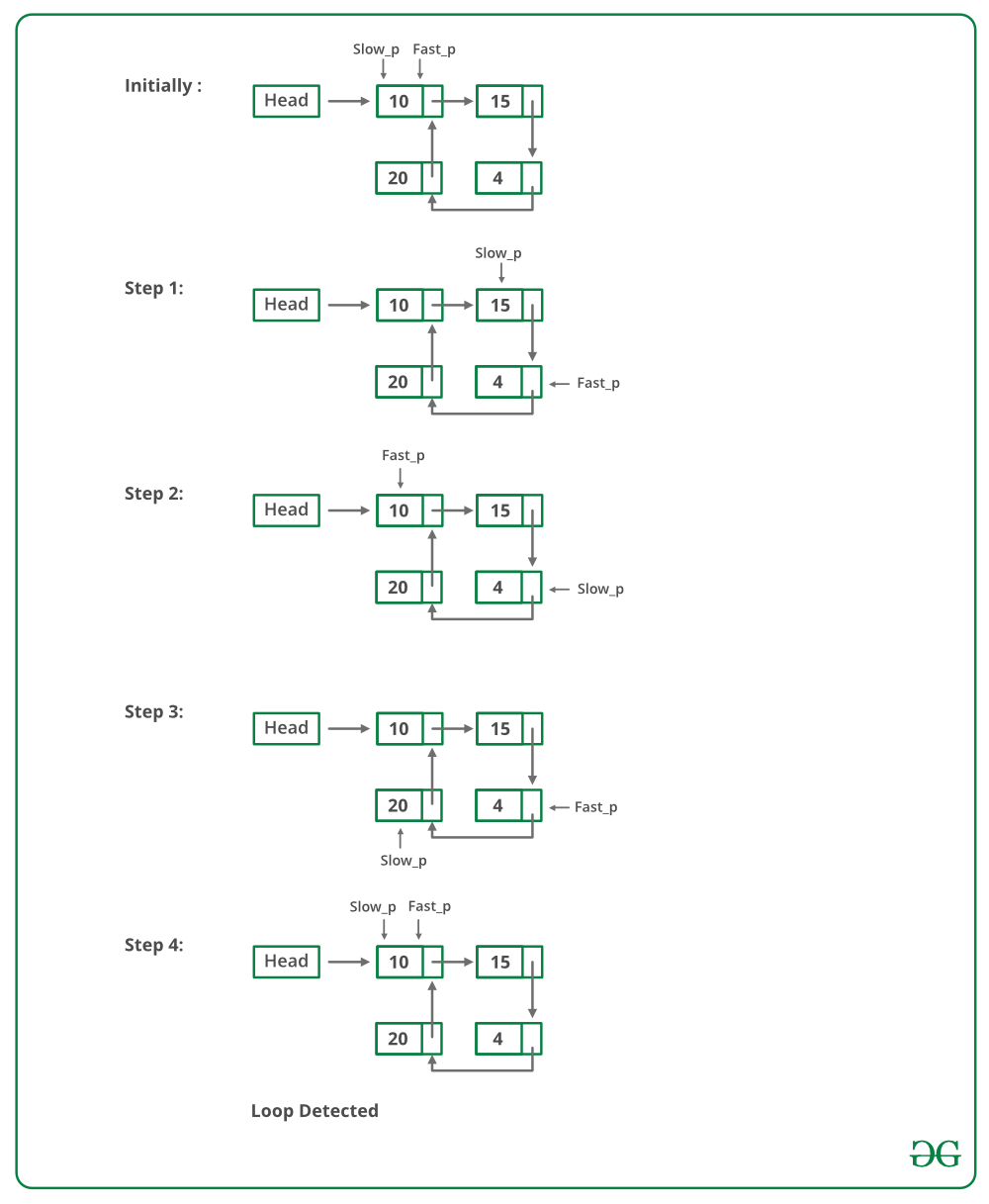# C Program For Detecting Loop In A Linked List

• Last Updated : 11 Dec, 2021

Given a linked list, check if the linked list has loop or not. Below diagram shows a linked list with a loop.Solution: Floyd’s Cycle-Finding Algorithm
Approach: This is the fastest method and has been described below:

• Traverse linked list using two pointers.
• Move one pointer(slow_p) by one and another pointer(fast_p) by two.
• If these pointers meet at the same node then there is a loop. If pointers do not meet then linked list doesn’t have a loop.

The below image shows how the detectloop function works in the code:Implementation of Floyd’s Cycle-Finding Algorithm:

## C

 `// C program to detect loop in a linked list``#include ``#include `` ` `/* Link list node */``struct` `Node {``    ``int` `data;``    ``struct` `Node* next;``};`` ` `void` `push(``struct` `Node** head_ref, ``int` `new_data)``{``    ``/* allocate node */``    ``struct` `Node* new_node``        ``= (``struct` `Node*)``malloc``(``sizeof``(``struct` `Node));`` ` `    ``/* put in the data  */``    ``new_node->data = new_data;`` ` `    ``/* link the old list off the new node */``    ``new_node->next = (*head_ref);`` ` `    ``/* move the head to point to the new node */``    ``(*head_ref) = new_node;``}`` ` `int` `detectLoop(``struct` `Node* list)``{``    ``struct` `Node *slow_p = list, *fast_p = list;`` ` `    ``while` `(slow_p && fast_p && fast_p->next) {``        ``slow_p = slow_p->next;``        ``fast_p = fast_p->next->next;``        ``if` `(slow_p == fast_p) {``            ``return` `1;``        ``}``    ``}``    ``return` `0;``}`` ` `/* Driver program to test above function*/``int` `main()``{``    ``/* Start with the empty list */``    ``struct` `Node* head = NULL;`` ` `    ``push(&head, 20);``    ``push(&head, 4);``    ``push(&head, 15);``    ``push(&head, 10);`` ` `    ``/* Create a loop for testing */``    ``head->next->next->next->next = head;`` ` `    ``if` `(detectLoop(head))``        ``printf``(``"Loop found"``);``    ``else``        ``printf``(``"No Loop"``);``    ``return` `0;``}`
Output
`Loop found`

Complexity Analysis:

• Time complexity: O(n).
Only one traversal of the loop is needed.
• Auxiliary Space:O(1).
There is no space required.

How does above algorithm work?
Please See : How does Floyd’s slow and fast pointers approach work?